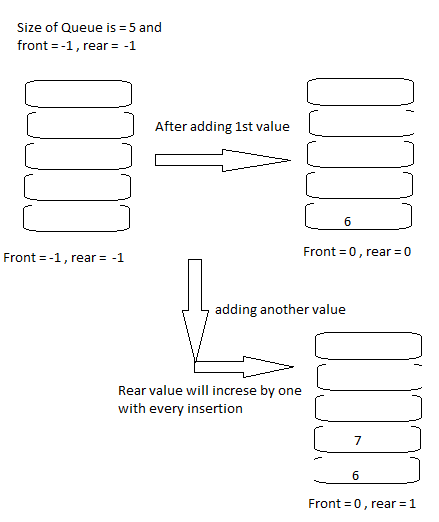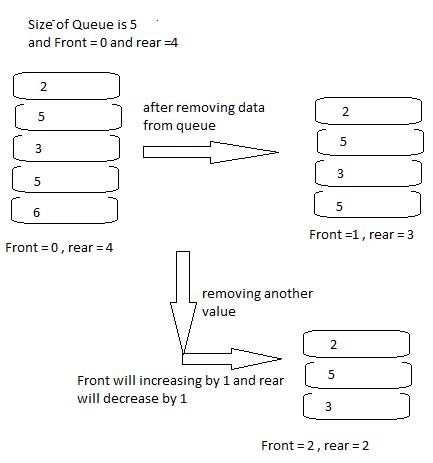Queue is another data structure that store and organize data into memory.

In this chapter we will discuss about queue features, queue operations and the java implementation of the algorithm.

If you are not aware about the basics of data structure, please click on the link given below.  Introduction of Data Structures

1.
Queue comes in non-primitive category and it is Linear Lists type data structure.It stores data elements in sequential manner.

2.

Queue works with predefined capacity that is way it’s comes in static data structure’s category.

3.

Every element comes in queue from the front and goes out first as well. Queue known as FIFO (First in First out).

Example: Like queue in real life for any match, movie etc where who comes first will get response first.

4.

In queue insertion happens from front and deletion happens from rear (end). Insertion process known as Enqueue and deletion process known as Dequeue.

Operations Of Queue -

enqueue()

This operation is to insert data element into queue from Front.

dequeue()

This operation is to remove data element from queue from Rear.

isEmpty()

This operation is to check underflow state of queue either queue is empty or not.

isFull()

This operation is to check overflow state of queue either queue is full or not.

peek()

This operation is to get all element data from queue, it does not remove data from queue.

How Queue Works – Implementation –

Enqueue/Insert Implementation

We are assuming that we have list of 5 elements and we want to insert or store data element into queue, let’s explain by using pseudo codes.

1.

Queue size is 5 so before enqueue any data we must check that queue is full or not if not full then insert data into memory. If queue is full then will show Overflow message to user.

``````
maxSize =5

front = -1 // first element of queue, initially set as -1

rear = -1 // last element of queue, initially set as -1

If ( rear = maxSize )

then  “Queue is Overflow, can’t add more element”
``````
2.

If queue is not full then insert data into queue, identify this first data element by name front and rear, when next element comes in queue then rear will increase by 1.
``````
Otherwise

rear = rear + 1

if ( front = -1 )

front = front + 1

Otherwise

Queue[rear] = element``````Queue Enqueue Operation. ByTechAchievers.com

Dequeue/Remove Implementation

We are assuming that we have 5 elements in queue and we want to insert or remove data element from queue, let’s explain by using pseudo codes.

1.

Before removing data from queue, first check queue is empty or not, if queue is empty then will show underflow message to user.
``````
If ( front = -1 )

then “Queue is underflow, can’t remove element”
``````

2.
If queue is not empty then replace front  value with front+1, now queue will have new front value and rear value will be decrease by 1 so that queue list is getting short, When there is only one data element remaining in queue then it would be rear and first element, after removing it front and rear both become -1.
``````
Otherwise

If (rear = front ) then

front = -1

rear = – 1

otherwise

Queue[front] = Queue[front+1]``````Queue Dequeue Operation. ByTechAchievers.com

isEmpty Implementation

As the function name suggest, isEmpty() method checks data availability in queue. This is Boolean method i.e. it returns true if queue is empty otherwise returns false.

While removing data element from queue, rear decrease by 1,

when rear become equals to front then both value will decrease by 1 and queue will be empty.
``````
If ( front = -1 )

then “Queue is empty ”
``````

isFull Implementation

As the function name suggest, isFull() method checks storage limit in queue or either queue has reached to its storage limit or not.

While adding data elements in queue, first data value would be first and rear element of queue, both will increase by 1, when anther value inserts then rear value increase by 1 until it reaches to maxSize of queue.
``````
if ( rear = maxSize )

then “ Queue is full “
``````

peek Implementation

peek() method is to return front element of queue, first checks if queue is not empty then get value of the queue.

This will return the value of front element of queue.
``````
if ( front = -1 )

then “ Queue is empty “

otherwise

frontValue = queue [front]``````

Queue Implementation In Java –

Let’s create a java program for queue data structure implementation, again I mention here that data structure is a way to store and organize data values into memory, there are many data structures are available with their features and benefits, we select them as per our requirement, Queue is one of them.

Let’s assume that we have list of students data and we want to store and organize them in queue First-in-First-out manner, so that every entry would be on rear.

``````
import java.util.ArrayList;

class ````Student```` {

String stud_name;

Integer rollNo;

``````Student(String sName, Integer rNo, String grd) {
``````
stud_name = sName;

rollNo = rNo;

````}````

}

interface ````Queue ````{

public void enqueue(Object data);

public void dequeue();

public boolean isEmpty();

public boolean isFull();

public Object peek();

}

class ````QueueImplementation ``implements ``Queue ````{

``````//showing front and rear of queue memory
``````

private static int front = -1;

private static int rear = -1;

private  Object[] queueList = null;

private static int queue_size_limit = 0;

````//constructer to set queue size limit````

``````QueueImplementation (int maxQueueSize) {
``````

queue_size_limit = maxQueueSize;

````/* memory allocation for maxQueueSize to store object data */````

queueList = new Object[queue_size_limit];

````}````

``````// Enqueue method to insert data element into queue
``````

````public void enqueue(Object data) {````

if ( isFull() ) {

System.out.println("Can't insert more element.");

}

else {

rear = rear + 1;

if ( front == -1 ) {

front = front + 1;

queueList[front] = data;

}

queueList[rear] = data;

}

````}```` //end of enqueue method

``````//Dequeue to remove data element from queue
``````

````public void dequeue() {````

if (isEmpty()) {

System.out.println("Can't remove more element.");

}

else {

if (rear == front ) {

front = -1;

rear = -1;

}

front = front + 1;

}

````}```` //end of dequeue method

````//isEmpty to check queue is empty or not````

``````public boolean isEmpty() {
``````

if (front == -1) {

System.out.println(" Queue is in underflow(empty) state. ");

return true;

````}````

return false;

````}```` // end of isEmpty method

````// isFull to check queue memory is full or not````

````public boolean isFull() {````

if ( rear == queue_size_limit-1 ) {

System.out.println(" Queue is in overflow(full) state. ");

return true;

}

return false;

````}```` // end of isFull method

````//peek method is to get top value from queue````

````public Object peek() {````

if (isEmpty()) {

System.out.println("Can't get queue element.");

return null;

}

return queueList[front];

} // end of peek method

````}```` // end of implementation class

public class ````QueueSampleProgram ````{

`````` public static void main(String[] args) {
``````

System.out.println("Creating student data ... ");

Student s1 = new Student ("Student1",1,"A");

Student s2 = new Student ("Student2",2,"B");

Student s3 = new Student ("Student3",3,"C");

Student s4 = new Student ("Student4",4,"D");

Student s5 = new Student ("Student5",5,"E");

System.out.println("Enqueue student data into queue ... ");

QueueImplementation queueImpl = new QueueImplementation(5);

queueImpl.enqueue(s1);

queueImpl.enqueue(s2);

queueImpl.enqueue(s3);

queueImpl.enqueue(s4);

queueImpl.enqueue(s5);

System.out.println("Get front of queue ...");

Student studData = (Student) queueImpl.peek();

System.out.println("Student data values :"+studData.stud_name+","+studData.rollNo+","+studData.grade);

System.out.println("Dequeue student data from queue ... ");

queueImpl.dequeue();

System.out.println("Get front of queue ...");

studData = (Student) queueImpl.peek();

System.out.println("Student data values :"+studData.stud_name+","+studData.rollNo+","+studData.grade);

````}````

}
``````
In the given example you can better understand interface layer where queue interface that has all queue methods.

The "queueImplementation" class that is implementing all the methods.

Here we are storing student object into queue memory, so we have student class, from main method we pass student object to the methods and all queue methods are dealing with student object.

Queue implementation layer can be used for any object, we can reuse queue data structure implementation for any other object.